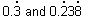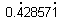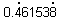HOME MATH DICTIONARY DOWNLOAD FEEDBACK DISCLAIMER
 Question: What is Recurring Decimal ? Answer: A decimal where the pattern of digits after the decimal point keeps repeating itself is said to be recurring. It may be a single digit repeating in 0.333333333333333.... or a repeating sequence as with 0.2382382382338238...., where the 238 is repeated endlessly. To indicate such a repetition a dot is placed over the first and last digit of the recurring sequence, so the above two numbers are written asrespectively. Most division if continued far enough turn out to give recurring decimals. For example, 3 ÷ 7 = 0.428571428571... =and 6 ÷ 13 = 0.461538461538 …. =For example, the decimal representation of 1/3 = 0.3333333... (spoken as 0.3 repeating) becomes periodic just after the decimal point, repeating the single-digit sequence 3 indefinitely. A somewhat more complicated example is 3227/555 = 5.8144144144..., where the decimal representation becomes periodic at the second digit after the decimal point, repeating the sequence of digits 144 forever.